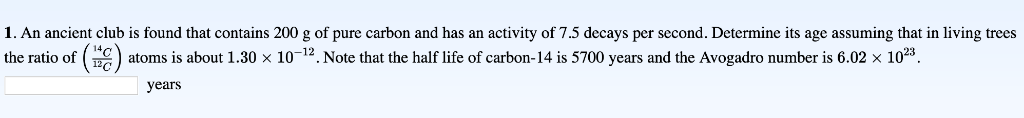# 1. An ancient club is found that contains 200 g of pure carbon and has an...

###### Question:1. An ancient club is found that contains 200 g of pure carbon and has an activity of 7.5 decays per second. Determine its age assuming that in living trees the ratio of (14C12C)(14C12C) atoms is about 1.30×10−121.30×10−12. Note that the half life of carbon-14 is 5700 years and the Avogadro number is 6.02×10236.02×1023.

#### Similar Solved Questions

##### You decide to build a small generator by rotating a coiled wire inside a static magnetic...
You decide to build a small generator by rotating a coiled wire inside a static magnetic field of 0.34 T. You construct the apparatus by coiling wire into 3 loops of radius 0.17 m. If the coils rotate at 2.0 revolutions per second and are connected to a device with 140Ω resistance, calculate t...
##### (7x9-5x'+x?-4) =(x-2)=
(7x9-5x'+x?-4) =(x-2)=...
##### 3. A rectangular channel has the following properties: b=5m, So=0.005, n=0.017. The flow rate in the...
3. A rectangular channel has the following properties: b=5m, So=0.005, n=0.017. The flow rate in the channel is 6 m3/sec. At a location A, the depth is 0.65 m, and at another location B, the depth is 0.9 m. (a) Classify the free-surface profile between A and B and (b) Determine the distance between ...
##### An object moving along the x-axis experiences a force of 55 N, which causes it to...
An object moving along the x-axis experiences a force of 55 N, which causes it to increase its velocity from 10.0 m/s to 22.6 m/s in a time interval of 4.2 s. What is the mass of this object? O 9.8 kg O 4.4 kg O 23.1 kg O 10.2 kg O 18.3 kg...